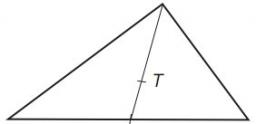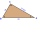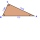# Median

The median of the triangle NOP is away from vertex P 95 cm. Calculate the length of the median, which start at P.

t =  142.5 cm

### Step-by-step explanation:Did you find an error or inaccuracy? Feel free to write us. Thank you!Tips to related online calculators
Check out our ratio calculator.

#### You need to know the following knowledge to solve this word math problem:

We encourage you to watch this tutorial video on this math problem:

## Related math problems and questions:

• MedianIn triangle ABC is given side a=10 cm and median ta= 13 cm and angle gamma 90°. Calculate length of the median tb.
• Median in right triangleIn the rectangular triangle ABC has known the length of the legs a = 15cm and b = 36cm. Calculate the length of the median to side c (to hypotenuse).
• Center of gravity and medianIn the isosceles triangle ABC, the center of gravity T is 2 cm from the base AB. The median parallel to the AB side measures 4 cm. What is the area of the ABC triangle?
• Medians in right triangleIt is given a right triangle, angle C is 90 degrees. I know it medians t1 = 8 cm and median t2 = 12 cm. .. How to calculate the length of the sides?
• The right triangleThe right triangle ABC has a leg a = 36 cm and an area S = 540 cm2. Calculate the length of the leg b and the median t2 to side b.
• Inscribed circleXYZ is right triangle with right angle at the vertex X that has inscribed circle with a radius 5 cm. Determine area of the triangle XYZ if XZ = 14 cm.
• Chord of triangleIf the whole chord of the triangle is 14.4 cm long, how do you calculate the shorter and longer part?
• The triangleThe triangle has sides 5 cm long, 5 cm, 8 cm long. What is the area of the triangle?
• RectangularRectangular triangle KLM with right angle at vertex L, angle beta at vertex K and angle alpha at vertex M. Angle at vertex M = 65°, side l = 17.5 cm. Use Pythagorean theorems and trigonometric functions to calculate the lengths of all sides and the angle
• Base of an isosceles triangleCalculate the size of the base of an isosceles triangle, the height is 5 cm and the length of the arm is 6.5 cm. What is the perimeter of this triangle?
• Right angleIn a right triangle ABC with a right angle at the apex C, we know the side length AB = 24 cm and the angle at the vertex B = 71°. Calculate the length of the legs of the triangle.
• RectangleThe length of the rectangle are in the ratio 5:12 and the circumference is 238 cm. Calculate the length of the diagonal and area of rectangle.
• CalculateCalculate the height to the base of the isosceles triangle ABC if the length of the base is c = 24cm and the arms have a length b = 13cm.
• In aIn a triangle, the aspect ratio a: c is 3: 2, and a: b is 5: 4. The perimeter of the triangle is 74cm. Calculate the lengths of the individual sides.
• Similar trianglesThe triangles ABC and XYZ are similar. Find the missing lengths of the sides of the triangles. a) a = 5 cm b = 8 cm x = 7.5 cm z = 9 cm b) a = 9 cm c = 12 cm y = 10 cm z = 8 cm c) b = 4 cm c = 8 cm x = 4.5 cm z = 6 cm
• The truncatedThe truncated rotating cone has bases with radii r1 = 8 cm, r2 = 4 cm and height v = 5 cm. What is the volume of the cone from which the truncated cone originated?
• Rectangular triangle PQRIn the rectangular triangle PQR, the PQ leg is divided by the X point into two segments of which longer is 25cm long. The second leg PR has a length 16 cm. The length of the RX is 20 cm. Calculate the length p of side RQ. The result is round to 2 decimal